Reach Us+32-10-28-02-25
The Spaces of Entire Function of Finite Order | OMICS International
Journal of Physical Mathematics
All submissions of the EM system will be redirected to Online Manuscript Submission System. Authors are requested to submit articles directly to Online Manuscript Submission System of respective journal.

# The Spaces of Entire Function of Finite Order

Malyutin KG1* and Studenikina IG2

1Department of Mathematics, Southwest State University, Russia.

2Department of Mathematics, Sumy State University, Ukraine.

*Corresponding Author:
Malyutin KG
Professor
Department of Mathematics
Southwest State University, Russia
Tel: 800-642-0684
E-mail: [email protected]

Received Date: June 03, 2016; Accepted Date: July 26, 2016; Published Date: August 01, 2016

Citation: Malyutin KG, Studenikina IG (2016) The Spaces of Entire Function of Finite Order. J Phys Math 7:190. doi:10.4172/2090-0902.1000190

Copyright: © 2016 Malyutin KG, et al. This is an open-access article distributed under the terms of the Creative Commons Attribution License, which permits unrestricted use, distribution, and reproduction in any medium, provided the original author and source are credited.

Visit for more related articles at Journal of Physical Mathematics

#### Abstract

This paper is a continuation of the research of the first author. We consider the linear topology space of entire functions of a proximate order and normal type with respect to the proximate order. We obtain the form of continuous linear functional on this space.

#### Keywords

Entire function; Proximate order; Normal type; Continuous linear functional

#### Introduction

This paper is a continuation of the research  where the linear topology space of entire functions of a proximate order and normal type, less than or equal σ, with respect to the proximate order were considered. We introduce the necessary definitions. A function ρ(r), defined on the ray (0,∞) and satisfying the Lipschitz condition on any segment [a, b]⊂ (0,∞) that satisfies the conditions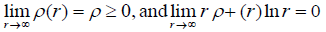This is called a proximate order.

A detailed exposition of the properties of proximate order can be found [2,3]. In this paper we use the notation V (r)=rρ(r). We will assume that V (r) is an increasing function on (0,∞) and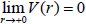.

We now formulate some simple property of proximate order that we shall need frequently .

For r →∞ and 0 < a ≤ k ≤ b < ∞ asymptotic inequality holds uniformly in k.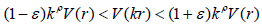(1)

Let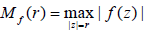. If for the entire function f(z) the quantity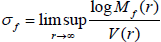Is different from zero and infinity, then ρ(r) is called of a proximate order of the given entire function f(z) and σf is called the type of the function f(z) with respect to the proximate order ρ(r).Let ρ(r) be a proximate order, limx→∞ ρ(r)= ρ ≥0. A single valued function f(z) of the complex variable z is said to belong to the space [ρ(r),¥) if f(z) has the order less than ρ(r) or equal ρ(r) but in this case type less than ¥. A sequence of functions {fn(z)} from [ρ(r),¥) converges in the sense of [ρ(r),¥) if

(i) It converges uniformly on compacts, (ii) there exists β<1 such that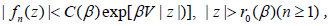where r0(β) does not depend on (n ≥ 1). For a suitable C(β), which does not depend on n, for all z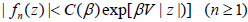(2)

The space [ρ(r),¥) is the linear topology space with sequence topology. Furthermore, a single valued function f(z) of the complex variable z is said to belong to the space [ρ(r), p] if f(z) has the order less than ρ(r) or equal ρ(r) but in this case type less than or equal p. A sequence of functions {fn(z)} from [ρ(r), p] converges in the sense of [ρ(r), p] if (i) it converges uniformly on compacts, (ii) for all ε > 0 there exists r0(ε) does not depend on n such that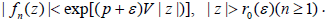The space [ρ(r), p] is also the linear topology space with sequence topology. We introduce the function φ(t) defined to be the unique solution of the equation t=V (r). So

φ(V (t))=t. (3)

Theorem 1.1 ([2, Theorem 2', p.42])

The type σf of the entire function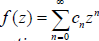with the proximate order ρ(r) (ρ > 0) is given by the equation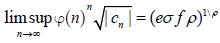(4)

Let ρ > 0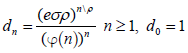For a function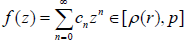we associate the function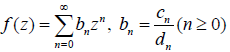(5)

It is regular, in any case in the circle |z| < 1 . Fact mapping function f(z) of [ρ(r), p] to the function F(z) as indicated above will be celebrating a record f(z) ~F(z).

In  it is proved the following two theorems.

Theorem 1.2

In order to be a sequence {fn(z)} of functions from [ρ(r), p] to converge in the sense of [ρ(r), p] necessary and sufficient that the sequence {fn(z)} (fn(z)~Fn(z)) converges uniformly inside the disk |z| < 1.

Theorem 1.3

Continuous linear functional l on the space [ρ(r), p] has the form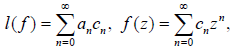(6)

Where the quantities an satisfy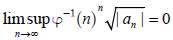(7)

The following is our main result.

Theorem 1.4

Continuous linear functional l on the space [ρ(r),¥ has the form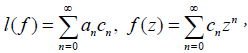(8)

Where the quantities an satisfy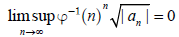(9)

#### The Space of Entire Functions [ρ(r),¥)

We now prove the theorem 1.4. Let l(f) be a continuous linear functional on the space [ρ(r),¥). Set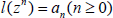Let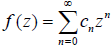be a function in [ρ(r),¥). Since the series converges in the sense of [ρ(r),¥) then, by continuous of the functional,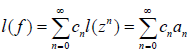Hence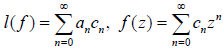(10)

Take an arbitrary finite p>0. Functional l(f) is, in particular, continuous linear functional on the space [ρ(r), p]. By theorem 1.3, the condition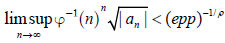But p is arbitrary, hence,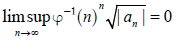We now verify that if the condition (9) then the functional (10) is continuous linear functional on the space [ρ(r),¥). Let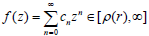By theorem 1.1,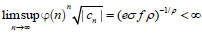. Then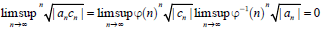And then the series (10) converges.

Let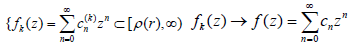if k¥ and let l satisfies (9). By (2), there exists β > 0 such that {{fk(z)}, f(z)}Ì[r(r), β] in the sense [ρ(r), β]. By (9) and (8) l is continuous linear functional on the space [ρ(r), β]. Then l(fk)→l l(f) if k¥. Therefore l is continuous linear functional on the space [ρ(r),¥).

#### Space of Entire Functions Eρ(r)

We now consider the space of entire functions Eρ(r) which have a proximate orders less then ρ(r). A proximate order ρ1(r) less then ρ(r) if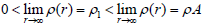aingle valued function f(z) of the complex variable z is said to belong to the space Eρ(r) if f(z) has the order less than ρ(r).

A sequence of functions {fn(z)} from Eρ(r) converges in the sense of Eρ(r) if (i) it converges uniformly on compacts, (ii) there exists proximate order ρ1(r),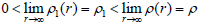such that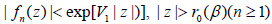(11)

where r0(β) does not depend on (n ≥ 1), V1(r)=rr1(r).The space Eρ(r) is the linear topology space with sequence topology. A continuous linear functional l(f) on the space Eρ(r) has the form (8). Let us find the conditions that satisfy the values an. The functional l(f) is in particular continuous linear functional on the space [ρ1(r),¥) for all proximate order ρ1(r),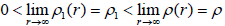. Therefore, by theorem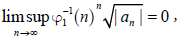(12)

where φ1(t) defined to be the unique solution of the equation t=V1(r). From this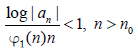So ρ1(r) is arbitrary less then ρ(r) that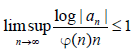(13)

Contrary, let the condition (13) is true and ρ1(r) is arbitrary less than ρ(r). So φ1(n) > φ(n), n > n0, thatTherefor the condition (12) is true and l(f) is continuous linear functional on the space [ρ(r),¥). So ρ1(r) is arbitrary less then, ρ(r) that l(f) is continuous linear functional on the space Er(r).

Theorem 3.1

Continuous linear functional l on the space E ρ(r) has the form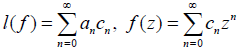Where the quantities an satisfy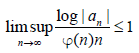Remark: The case of the spaces [ρ,¥) and Er, where ρ(r)=ρ > 0, considered A.F Leont’ev .

#### Conclusion

The linear topology space of entire functions of a proximate order and normal type with respect to the proximate order is considered. We obtain the form of continuous linear functional on this space through our work.

#### References

Select your language of interest to view the total content in your interested language

### Article Usage

• Total views: 8977
• [From(publication date):
September-2016 - Nov 15, 2019]
• Breakdown by view type
• HTML page views : 8830Can't read the image? click here to refresh
###### Peer Reviewed Journals

Make the best use of Scientific Research and information from our 700 + peer reviewed, Open Access Journals

### Clinical & Medical Journals

International Conferences 2019-20

Meet Inspiring Speakers and Experts at our 3000+ Global Annual Meetings

Top